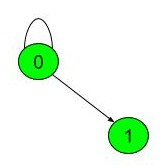Detect cycle in a directed graph
##### Submissions: 53669   Accuracy: 30.19%   Difficulty: Medium   Marks: 4

Given a Directed Graph. Check whether it contains any cycle or not.\

Input: The first line of the input contains an integer 'T' denoting the number of test cases. Then 'T' test cases follow. Each test case consists of two lines. Description of testcases is as follows: The First line of each test case contains two integers 'N' and 'M'  which denotes the no of vertices and no of edges respectively. The Second line of each test case contains 'M'  space separated pairs u and v denoting that there is an uni-directed  edge from u to v .

Output:
The method should return 1 if there is a cycle else it should return 0.

You don't need to read input or print anything. Your task is to complete the function isCyclic which takes the Graph and the number of vertices and inputs and returns true if the given directed graph contains a cycle. Else, it returns false.

Expected Time Complexity: O(V + E).
Expected Auxiliary Space: O(V).

Constraints:
1 <= T <= 1000
1<= N,M <= 1000
0 <= u,v <= N-1

Example:
Input:

3
2 2
0 1 0 0
4 3
0 1 1 2 2 3
4 3
0 1 2 3 3 2
Output:
1
0
1

Explanation:
Testcase 1:
In the above graph there are 2 vertices. The edges are as such among the vertices.From graph it is clear that it contains cycle.

#### ** For More Input/Output Examples Use 'Expected Output' option **

Contributor: Harshit Sidhwa
Author: Shubham Joshi 1

If you have purchased any course from GeeksforGeeks then please ask your doubt on course discussion forum. You will get quick replies from GFG Moderators there.